Latest Banking jobs   »   Reasoning Ability Quiz For IBPS RRB...

# Reasoning Ability Quiz For IBPS RRB PO, Clerk Prelims 2021- 4th May

Directions (1-5): In each of the questions below are given some statements followed by two conclusions. You have to take the given statements to be true even if they seem to be at variance with commonly known facts. Read all the conclusions and then decide which of the given conclusions logically follows from the given statements disregarding commonly known facts.
(a) If only conclusion I follows.
(b) If only conclusion II follows.
(c) If either conclusion I or II follows.
(d) If neither conclusion I nor II follows.
(e) If both conclusions I and II follow.

Q1. Statements: Only a few China is America. No America is India. All India is Europe.
Conclusions:
I. All Europe can be America.
II. Some China is not India.

Q2. Statements: Only a few phone is busy. Only busy is online. Some busy is connection.
Conclusions:
I. Some connection can be phone.
II. Some phone can be online.

Q3. Statements: All pink is blue. All blue is purple. Only a few pink is white.
Conclusions:
I. All white can be purple.
II. Some white can be blue.

Q4. Statements: No metal is lock. Some lock is key. Only a few metal is iron.
Conclusions:
I. All iron can be metal.
II. All key can never be metal.

Q5. Statements: Only a few series is episode. Only a few episode is free. All free is subscription.
Conclusions:
I. Some series is free.
II. Some subscription can be episode.

Directions (6-10): In each of the questions below are given some statements followed by two conclusions. You have to take the given statements to be true even if they seem to be at variance with commonly known facts. Read all the conclusions and then decide which of the given conclusions logically follows from the given statements disregarding commonly known facts.

Q6. Statements: All train is plane. All plane is bus. Some bus is auto.
Conclusions:
I. Some auto is plane.
II. No auto is plane.
(a) If only conclusion I follows.
(b) If only conclusion II follows.
(c) If either conclusion I or II follows.
(d) If neither conclusion I nor II follows.
(e) If both conclusions I and II follow.

Q7. Statements: All pink is blue. All blue is purple. No blue is white.
Conclusions:
I. Some purple can be white.
II. Some pink can be white.
(a) If only conclusion II follows.
(b) If only conclusion I follows.
(c) If neither conclusion I nor II follows.
(d) If either conclusion I or II follows.
(e) If both conclusions I and II follow.

Q8. Statements: No amazon is snapdeal. All snapdeal is flipkart. No flipkart is ebay.
Conclusions:
I. Some amazon is flipkart.
II. All snapdeal can be ebay.
(a) If either conclusion I or II follows.
(b) If only conclusion I follows.
(c) If both conclusion I and II follows.
(d) If neither conclusion I nor II follows.
(e) If Only conclusion II follow.

Q9. Statements: Some orange is pear. Some pear is apple. Some apple is mango.
Conclusions:
I. Some mango can be orange.
II. Some pear is not apple.
(a) If only conclusion II follows.
(b) If both conclusion I and II follows
(c) If neither conclusion I nor II follows.
(d) If either conclusion I or II follows.
(e) If only conclusion I follows.

Q10. Statements: No Bihar is Gujarat. No Gujarat is Bengal. All Gujarat is Kerala.
Conclusions:
I. Some Kerala can be Bihar.
II. All Bengal can be Kerala.
(a) If both conclusion I and II follows.
(b) If only conclusion I follows.
(c) If neither conclusion I nor II follows.
(d) If either conclusion I or II follows.
(e) If only conclusion II follows.

Directions (11-12): Read the following information carefully and answer the question which follows:
IF ‘A × B’ means ‘A is son of B’
IF ‘A + B’ means ‘A is daughter of B’
IF ‘A ÷ B’ means ‘A is wife of B’
IF ‘A – B’ means ‘A is Father of B’

Q11. What will come in the place of the question mark, to establish that M is mother of J in the expression?
‘J + K – L ? M’
(a) +
(b) ×
(c) –
(d) ÷
(e) Either (a) or (b)

Q12. Which of the following relations are true based upon the relations given in the question?
J ÷ K × L – M + N’?
(a) K is brother of N
(b) J is daughter-in-law of M
(c) J is daughter-in-law of N
(d) N is daughter of L
(e) None is true

Directions (13-14): Read the following information carefully and answer the questions given below:
There are seven members S, T, U, V, W, X and Y in a family among them only 3 females. S is married to V. W is grandson of V who has two children. U is sister in law of Y who is unmarried. X is brother in law of S who is mother in law of U.

Q13. How X is related to Y?
(a) Uncle
(b) Brother
(c) Mother
(d) Sister
(e) Grandson

Q14. Who among the following is the father of W?
(a) U
(b) T
(c) X
(d) Y
(e) None of these

Directions :(15) Study the following information carefully and answer the questions which follow–
‘P – Q’ means ‘P is father of Q’
‘P ÷ Q’ means ‘P is sister of Q’
‘P × Q’ means ‘P is mother of Q’
‘P + Q’ means ‘P is brother of Q’

Q15. Which of the following means ‘A is nephew of B’?
(a) A + C – B × K
(b) B ÷ H – A + D
(c) B ÷ G – A ÷ R
(d) B + T × A ÷ E
(e) None of these

Practice More Questions of Reasoning for Competitive Exams:

###### Study Plan for IBPS RRB PO/Clerk Prelims 2021

Solutions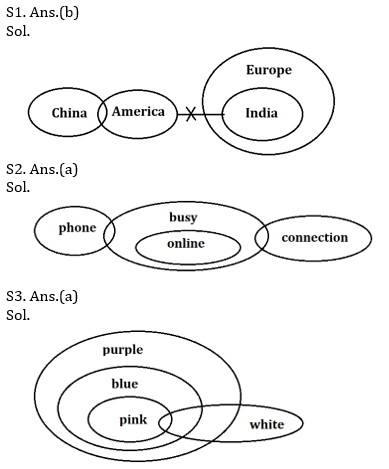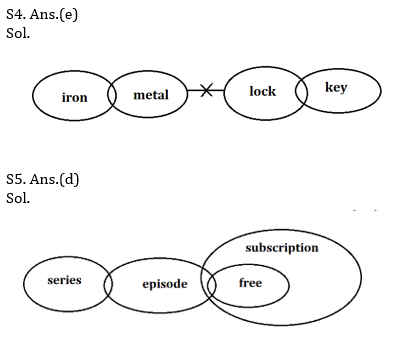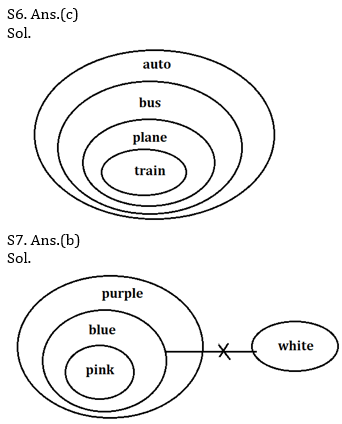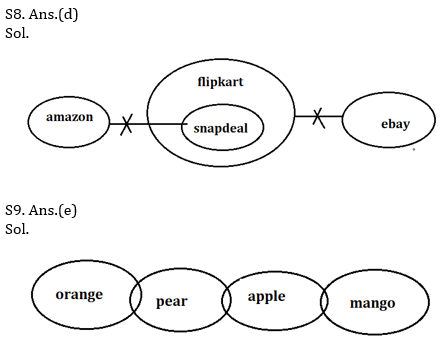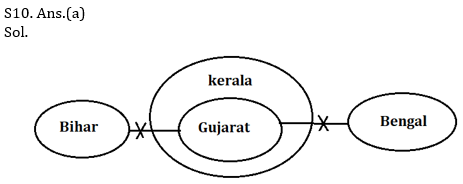S11. Ans.(e)
Sol. J is the daughter of K and K is the father of L. So for M to be mother of J, M should be the mother of L. Hence the question mark can be replaced by either × or +.

S12. Ans.(c)
Sol. Here L and N are husband and wife. M is their daughter, K is their son and J is their daughter-in-law. Hence only (c) is true.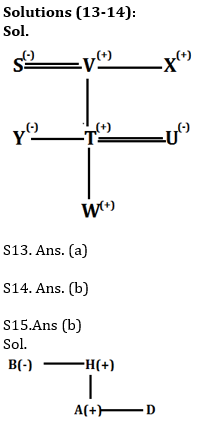#### Congratulations!Incorrect details? Fill the form again here

•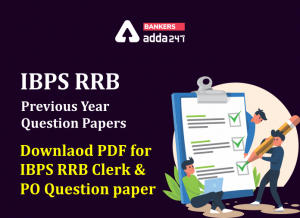IBPS RRB Previous Year Question Paper PD...
•Reasoning Ability Quiz For IBPS RRB PO C...
•Reasoning Ability Quiz For IBPS RRB PO C...
•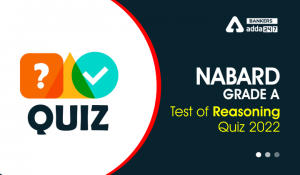Reasoning Ability Quiz For NABARD Grade ...
•Reasoning Ability Quiz For IBPS RRB PO C...
•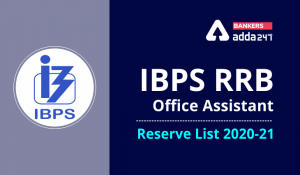IBPS RRB Clerk 2nd Reserve List 2022 Out...